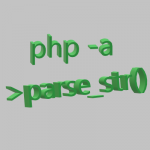## Transform Strings to Array in PHP

by

One of the wonderful things about a programing language is they include predefined functions. When trying to transform a string to a array there is a function for that.

PHP was the original template engine for making websites for the C programing language. PHP is often thought of as a sledge hammer of a programming language because with a minimal effort you can create interactive content on the web. Specially since there is a prebuilt function for almost anything.

Why convert a string to a array in the first place? Suppose you want to store a complex string in temporary storage, be it a file, or a temporary column in a row of a database.

The prebuilt function in php for converting strings to an Array is parse_str and you can try it out on a command line interactive shell on a system with php installed.

On a interactive shell e.g terminal run the following command:

``````
php -a
``````

To try out parse_str function you need to assign a string to a variable first. Let's make up a variable name:

parse_str takes in two variables inside the parenthesis. First variable is the string and the second variable is the output conversion of the string to a Array.

``````
parse_str(\$variableOne, \$outputVariable);
``````

You know about the input variable and the output variable because parse_str function is documented here

Sometimes you supposedly see open source code with excessive obfuscation to the point where variables are x's and y's and you wonder about the motive of the developer. Let's have a meaningful variable.

``````
\$storeStringForArray = "";
``````

Next we need to know the syntax (e.g. format) of the required string for the string to be transformed into a array using parse_str function.

The string should start with a ampersand. This way you can append to a existing string.

``````
\$storeStringForArray = "&";
``````

Most basic example of a string storing a key and a value would be:

``````
\$storeStringForArray = "&boxType[]=redBoxes";
``````

You may want to try storing a two dimensional array of items. To have the array be accessible using key value pairs.

To allow for the use of following code and output for the key boxType.

``````
echo \$outputArray[boxType];
``````

To set a key in the array, write a name with empty brackets followed by a ampersand. To set a value for each key, write equal and type the value.

You can try out the function by declaring following keys: stuff, boxType, and boxName.

Let's type in the string and store in variable named \$storeStringForArray

``````
php > \$storeStringForArray = "&stuff[]&boxType[]=redBoxes&boxName[]='box one'";
``````

Now you can use the parse_str function and give the function the variable you declared named \$storeStringForArray

The function needs to output the array to a variable and here you can name that variable \$outputArray.

``````
php > parse_str(\$storeStringForArray,\$outputArray);
``````

Now we can print the array using print_r function and hit enter.

``````
php > print_r(\$outputArray);
``````

The php interactive shell displays the following output.

``````
Array(    [stuff] => Array        (             =>        )    [boxType] => Array        (             => redBoxes        )    [boxName] => Array        (             => 'box one'        ))
``````

Now when you echo the boxType key, the php interactive shell displays the following.

``````
php > echo \$outputArray[boxType];redBoxes
``````

Well, there you have it. Objects are to JavaScript as Arrays are to PHP.

This type of clear concise information is usually buried in documentation and requires research with trial and error to uncover.